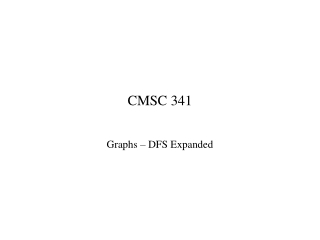DownloadDownload PresentationCMSC 341

# CMSC 341

Télécharger la présentation## CMSC 341

- - - - - - - - - - - - - - - - - - - - - - - - - - - E N D - - - - - - - - - - - - - - - - - - - - - - - - - - -
##### Presentation Transcript

1. CMSC 341 Graphs – DFS Expanded

2. Depth First Traversal with Finish Times dfs(Graph G) { for (each v  V) d[v] = 0 // d = discovery “time” time = 0 // “global” variable for (each v  V) if (d[v] = 0) // not discovered yet dfs (v) } dfs(Vertex v) { time = time + 1 d[v] = time // “discover” and mark v for(each vertex w adjacent from v) if (d[w] = 0) // w not discovered dfs(w) time = time + 1 f[v] = time // v is “finished” }

3. Edge Types • After DFS, edges can be classified into the following types: • tree edges -- a discovered vertex v1 encounters an undiscovered vertex v2; the edge between them is a tree edge • back edges -- a discovered vertex v1 encounters a discovered but unfinished vertex v2; the edge between them is a back edge. (Graph has a cycle if and only if there is a back edge.) • forward edges (directed graphs only) -- a discovered vertex v1 encounters a finished vertex v2 • cross edges (directed graphs only) -- a discovered vertex v1 encounters a finished vertex v2 and d[v1] > d[v2]

4. Edge Types (after DFS completion) • Condition Type of Edge (v1, v2)

5. Utility of Discovery/Finish Times • A graph contains a cycle if and only if it contains a back edge. • Finish times can be used to do a topological sort of a digraph (later). • Finish times can be used to find strongly connected components in a graph.